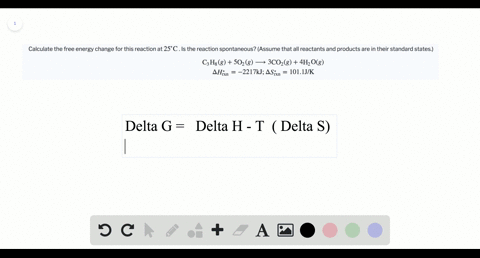### Calculate the free energy change for this reactio…

02:16
LP
Problem 44

Calculate the change in Gibbs free energy for each of the sets of $\Delta H_{\text { ren }}, \Delta S_{\text { rxn }}$ and $T$ given in Problem $42 .$ Predict whether or not each reaction is spontaneous at the temperature indicated. (Assume that all reactants and products are in their standard states.)

PART $\mathrm{A} : \Delta G^{\circ}=-48 \mathrm{kJ}$ and spontaneous
PART $\mathrm{B} : \Delta G^{\circ}=39 \mathrm{kJ}$ and nonspontaneous
PART $\mathrm{C} : \Delta G^{\circ}=142 \mathrm{kJ}$ and nonspontaneous
PART $\mathrm{D} : \Delta G^{\circ}=-157 \mathrm{kJ}$ and spontaneous

## Discussion

You must be signed in to discuss.

## Video Transcript

So this problem is going off of question 42 it wants you to calculate, gives free energy based on those values they give you and to state whether this reaction be spontaneous and spontaneous at that temperature so we can calculate gives for energy using this equation. Don't h modesty don't s. And since we have all those values given to us, we can just plug in and see what we get. So, for a delta H equals minus 95 killed Jules, don't that s equals by the 0.157 co jewels for Helen. And so what I did is the value was rigid, written in jewels, but to keep the units to the same, I went ahead and cover to this to kill Jules, but a batting about 1000 and then t physical of 2 98 So if you plug that in to your gives the question yet minus 95 minus imprint disease 2 98 terms negative. 0.157 And what you get is minus 40 eight point to kill Jules. And so determined where this reaction of spontaneous or not spontaneous you'll look at the sign of your Gibbs for energy. And since it's negative, it's spontaneous. And for this purpose, I was right, S or N s t o do know. Spontaneous, non spontaneous. So we do the same thing for B again, minus 95. Heard of age. Don't s also is the same. Negative 0.157 and kill Jules her coven. What? See, this time it is now a 55 Kevin. So you plug it in, like before in Delta G equal, this is zero minus 0.157 Delta G turns out to be 39 killer Jules. And because the sound is positive, this reaction is not spontaneous. Same thing for C. The H equals 95 killed. Jules don't s equals minus 0.157 Colegiales per coven. Remember, I'm dividing my 1000 to keep both dealt. Agent Delta s in the same scale of jewels or killer Jules and two equals 2 98 Kelvin, always take your units. Um, so don't g in this case is 1 41 0.78 killer Jules. And so this reaction This lastly I heard for D We have Delta H equals negative. 95 killed. Jules. Negative 95. Killer. Jules Don't s equals positive. 0.157 killed. Jules for Calvin T is equal to 3 98 Kelvin. And so you plugged it into Gives free energy equation and Delta G equals minus 1 57 killed, Jules. And because this sinus night different G, this reaction is spontaneous.